### Iterated Function Systems : Barnsley Fern

In his book, Fractals Everywhere, Michael Barnsley writes
IFSs provide models for certain plants, leaves, and ferns, by virtue of the self-similarity which often occurs in branching structures in nature.
The concept of Iterated Function Systems was first given by John Hutchinson in 1981 and was later popularized by Michael F. Barnsley. The fractal which we are going to discuss in this article is named Barnsley Fern due to the contributions of Barnsley in this field.

### What are IFSs?

Iterated Function Systems are a method of constructing fractals. They are called iterated because of the hundreds or thousands of iterations we perform on the image to get the final self repeating image i.e. the fractal. A method of rendering IFS fractals is called the chaos game which we will be discussing in this article. In this method random points are chosen and affine transformation functions are applied iteratively to those points which means the result of the current iteration becomes the input for the next iteration.

### What is an Affine Transformation?

An affine transformation is a function which is used to perform scaling, reflection, rotation, shear and many other operations on points. An affine transformation does not necessarily preserve the angle between two lines but a set of parallel lines remain parallel after an affine transformation is applied to them.
An affine transformation is generally applied in the form of a matrix. In the following section I'll explain this with an example.
Suppose we have a point (x0,y0) in the co-ordinate plane then to the affine transformation to get the resulting point (x1,y1) the affine transformation is applied as follows:where a,b,c,d,e and f are constants.
Let's take four points in 2D plane which represent a square with side 10 units: (0,0), (10,0), (0,10) and (10,10).
Now let's take affine transformation matrices with : a = 0, b = 1, c = 2, d =1, e = -10 and f = -10. The function can now be represented as:Now we feed all the four points of the square to this function one by one. For example when we input (10,10) we get (0,20) as the output. When we join all the four output points we get an image very different from the input square. The following image shows this transformation graphically. The blue square is the original input and the red parallelogram is the outcome of the transformation: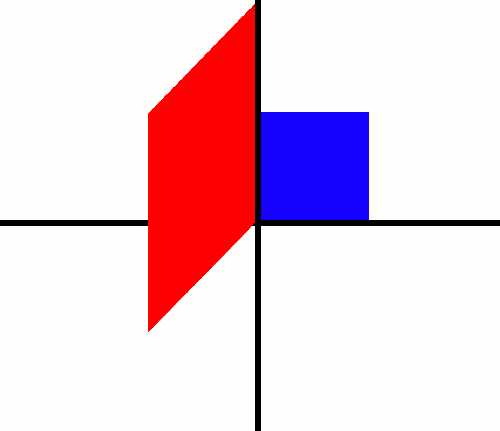This is how the affine transformation works.

### So, how are the IFS fractals rendered?

We first define more than one affine functions (four for the classic Barnsley fern). Then we use the chaos game to choose random points in the image and apply one of the four affine functions and feed the result of this iteration to the next iteration. We render each output of the functions with a specific color and we get the resultant fractal.
Each affine function is represented as ω(x) = A.x+ t where A is the transformation matrix, x is the point (x,y) and t is translation matrix.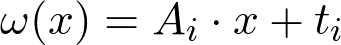So, we're ready with our four functions but how do we know when to use which function or how often a function is to be used?
The answer is, we associate another number with each function i.e. its probability. The following formula can be used to get rough probabilities for each function. These can however be modified to get better results.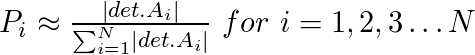where |det. Ai| is the absolute value of determinant of the matrix Ai and N is the number of functions used which is given by:
|det. Ai| = |a.d - b.c|
If, for some i, we get Pi=0 the we assign Pi a small positive value like 0.001.
While finally using the probabilities for rendering it should be noted that ∑ Pi=1
Now, we can use these probabilities to determine how often a function is used to plot the points.
The following four Affine functions are used for the classic Barnsley fern:
Functions a b c d e f Probability
f1 0.000 0.000 0.000 0.160 0.000 0.000 0.010
f2 0.850 0.040 -0.04 0.850 0.000 1.600 0.850
f3 0.200 -0.26 0.230 0.220 0.000 1.600 0.070
f4 -0.15 0.280 0.260 0.240 0.000 0.440 0.070

### The Pseudo Code

``````AffineFunctions f1,f2,f3,f4
Probabilities p1,p2,p3,p4
N = number of iterations
Point p = a random point with x and y є [0,1]
W,H = Width, Height of Image
for i from 0 to N:
P = Probability
if P < p1:
new_p = f1.transform(p)
else if P < p1+p2:
new_p = f2.transform(p)
else if P < p1+p2+p3:
new_p = f3.transform(p)
else:
new_p = f4.transform(p)
p = new_p
Translate Point p to image co-ordinates and render``````

### Java Implementation

Let's begin by implementing a point on 2D plane in Java.
``````package math.transform;
/**
* Point is a class which implements a point in 2D plane in Java.
*
* @author  Abdul Fatir
* @version 1.0
*/
public class Point {
// The X co-ordinate of the Point.
private double x;
// The Y co-ordinate of the Point.
private double y;
// Constructs a new Point with X = 0.0 and Y = 0.0
public Point() {
x = 0.0;
y = 0.0;
}
/**
* Constructs a new Point object.
* @param x The X co-ordinate
* @param y The Y co-ordinate
*/
public Point(double x, double y) {
this.x = x;
this.y = y;
}
// @return the X co-ordinate of the Point
public double getX() {
return this.x;
}
// @return the Y co-ordinate of the Point
public double getY() {
return this.y;
}
/**
* Sets the X co-ordinate to the given argument.
* @param x The X co-ordinate
*/
public void setX(double x) {
this.x = x;
}
/**
* Sets the Y co-ordinate to the given argument.
* @param y The Y co-ordinate
*/
public void setY(double y) {
this.y = y;
}
/**
* Translates the point with the passed values.
* @param dx The translatation in X.
* @param dy The translatation in Y.
*/
public void translate(double dx, double dy) {
x += dx;
y += dy;
}
}``````
Next we implement a 2x2 matrix in Java.
``````package math.transform;
/**
* Matrix2D is a class which implements a 2x2 matrix in Java.
*
* @author      Abdul Fatir
* @version   1.0
*
*/
public class Matrix2D {
/**
* The 2D array which represents the 2x2 matrix.
*/
private double[][] matrix=new double;
/**
* Constructs a new Matrix2D with all elements 0.
*/
public Matrix2D() {
matrix = 0.0;
matrix = 0.0;
matrix = 0.0;
matrix = 0.0;
}
/**
* Constructs a new Matrix2D with the passed elements.
* @param a The [0,0] element of the matrix
* @param b The [0,1] element of the matrix
* @param c The [1,0] element of the matrix
* @param d The [1,1] element of the matrix
*/
public Matrix2D(double a, double b, double c, double d) {
matrix = a;
matrix = b;
matrix = c;
matrix = d;
}
/**
* Constructs a new Matrix2D using the passed array.
* @param matrix The 2D array representing the matrix
*/
public Matrix2D(double[][] matrix) {
this.matrix = matrix;
}
/**
* Computes the determinant of the current matrix.
* @return The determinant of the current matrix
*/
public double determinant() {
return ((matrix*matrix)-(matrix*matrix));
}
}``````
Now, let us implement the Affine Transformation in Java.
``````package math.transform;
import math.transform.Point;
/**
* AffineTransformation is a class which implements affine transformation in Java.
*
* @author      Abdul Fatir
* @version   1.0
*
*/
public class AffineTransformation {
/**
* The transformation matrix i.e. a,b,c,d
*/
private double[][] transformation=new double;
/**
* The translation matrix i.e. e,f
*/
private double[][] translation=new double;
/**
* Constructs an AffineTransformation with all elements 0.
*/
public AffineTransformation() {
transformation = 0.0;
transformation = 0.0;
transformation = 0.0;
transformation = 0.0;
translation = 0.0;
translation = 0.0;
}
/**
* Constructs an AffineTransformation with given transformation matrix and translation matrix 0.
* @param transformation the transformation matrix i.e. a,b,c,d
*/
public AffineTransformation(double[][] transformation) {
this.transformation = transformation;
translation = 0.0;
translation = 0.0;
}
/**
* Constructs an AffineTransformation with given transformation matrix and translation matrix.
* @param transformation the transformation matrix i.e. a,b,c,d
* @param translation the translation matrix i.e. e,f
*/
public AffineTransformation(double[][] transformation, double[][] translation) {
this.transformation = transformation;
this.translation = translation;
}
/**
* Constructs an AffineTransformation with given a,b,c,d,e,f.
* @param a the [0,0] element of the transformation matrix
* @param b the [0,1] element of the transformation matrix
* @param c the [1,0] element of the transformation matrix
* @param d the [1,1] element of the transformation matrix
* @param e the [0,0] element of the translation matrix
* @param f the [1,0] element of the translation matrix
*/
public AffineTransformation(double a,double b,double c,double d,double e,double f) {
transformation = a;
transformation = b;
transformation = c;
transformation = d;
translation = e;
translation = f;
}
/**
* Transforms the passed point with the current AffineTransformation
* @return The transformed point
*/
public Point transform(Point point) {
double x = point.getX();
double y = point.getY();
double u = 0.0;
double v = 0.0;
u = transformation*x + transformation*y;
v = transformation*x + transformation*y;
u = u + translation;
v = v + translation;
return new Point(u,v);
}
/**
* @return a Matrix2D object of the transformation matrix.
*/
public Matrix2D getMatrix2D() {
return new Matrix2D(transformation,transformation,transformation,transformation);
}
/**
* @param transformations The AffineTransformations used.
* @return An array containing the probabilities to be used for each affine transformation
*/
public static double[] getProbabilities(AffineTransformation... transformations) {
double sum_of_determinants = 0;
double probabilities[] = new double[transformations.length];
for(AffineTransformation affine : transformations) {
sum_of_determinants+= Math.abs(affine.getMatrix2D().determinant());
}
int i = 0;
for(AffineTransformation affine : transformations) {
probabilities[i] = Math.abs(affine.getMatrix2D().determinant())/sum_of_determinants;
i++;
}
return probabilities;
}
}``````
Now that we have framed the skeleton of the Iterated function systems, we are ready to use them and create some beautiful ferns.
``````import math.transform.AffineTransformation;
import math.transform.Point;
import java.io.IOException;
import java.io.File;
import javax.imageio.ImageIO;
import java.awt.image.BufferedImage;
import java.awt.Color;
import java.awt.Graphics;
public class BarnsleyFern {
public static void main(String[] args) throws IOException {
// Defining the four affine transformations for the classic BarnsleyFern
AffineTransformation f1 = new AffineTransformation(0,0,0,0.16,0,0);
AffineTransformation f2 = new AffineTransformation(0.85,0.04,-0.04,0.85,0,1.6);
AffineTransformation f3 = new AffineTransformation(0.2,-0.26,0.23,0.22,0,1.6);
AffineTransformation f4 = new AffineTransformation(-0.15,0.28,0.26,0.24,0,0.44);
// Defining the color in which the fern will be drawn
Color color = Color.GREEN;
// Defining the Width and Height of the Image
int W=1600;
int H=1200;
// Creating a new blank RGB Image
BufferedImage image = new BufferedImage(W, H,BufferedImage.TYPE_3BYTE_BGR);
// Filling the whole image with black color
Graphics g=image.getGraphics();
g.setColor(Color.BLACK);
g.fillRect(0,0,W,H);
// Choosing the first point randomly using Math.random() which returns a value [0,1]
Point point = new Point(Math.random(),Math.random());
// Defining N which will govern our number of iterations
int N=W*H;
// Defining an array of length N which represents each pixel in the image
int pixels[] = new int[N];
// Iterating N*25 times
for(int i=0;i< N*25;i++) {
Point newpoint = new Point();
// Using a random number between [0,1] as the probability
double probability = Math.random();
// Transforming the point based on the probability
if(probability < 0.01) {
newpoint = f1.transform(point);
} else if(probability < 0.01 + 0.85) {
newpoint = f2.transform(point);
} else if(probability < 0.01 + 0.85 + 0.07) {
newpoint = f3.transform(point);
} else {
newpoint = f4.transform(point);
}
point = newpoint;
// Translating the point to the co-ordinates of the Image
int X = (int)(point.getX()*W/10) + W/2-1;
int Y = (H-1) - (int)(point.getY()*H/10);
// Setting the value of that specific pixel co-ordinate to the RGB value of our color
pixels[W*Y+X] = color.getRGB();
}
// Writing the RGB pixels array to the Image
image.setRGB(0,0,W,H,pixels,0,W);
//Saving the Image
ImageIO.write(image,"PNG", new File("barnsleyfern.png"));
}
}``````

#### Explaining the Code

Almost all of the code is pretty self explanatory give the comments however I would like to touch upon the following part which does the task of translating the point to image co-ordinates:
``````int X = (int)(point.getX()*W/10) + W/2-1;
int Y = (H-1) - (int)(point.getY()*H/10);``````
The output given by the affine transformations is within the range −2.1820 < x < 2.6558 and 0 ≤ y < 9.9983. So, in order to translate these values into pixel co-ordinates we require some computations. For example, the absolute range of x [−2.1820, 2.6558] is approximately 5 and that of y [0, 9.9983] is approximately 10.
We calculate the Y co-ordinate by multiplying `point.getY()` with Height of the image and then dividing it by 10. This will lead to:
`Y = point.getY()*H/10`
but this leads to the creation of an inverted image hence we subtract it from (H-1) to make the image upright. The -1 is used because image co-ordinates range from [0,H) leading to the final expression:
Y = (H-1) - (point.getY()*H/10)
The case of X is a bit more complex than this. First we multiply `point.getX()` with the Width of the image and divide it by 5 (the absolute range of x) but since the co-ordinates will come negative by the approach we need to add W/2 to translate the lowest pixel X co-ordinate to 0 thereby giving the formula:
`X = (point.getX()*W/5) + W/2-1`
The -1 is again used for the above mentioned reason. This formula however leads to a bloated image so we need to shrink it in X and hence we divide the whole equation by 2, leading to:
`X = (point.getX()*W/10) + W/4-1`
This creates a perfect image but shifts it to the left half so we add another W/4 to get the final formula:
`X = (point.getX()*W/10) + W/2-1`
The above code results in the following output: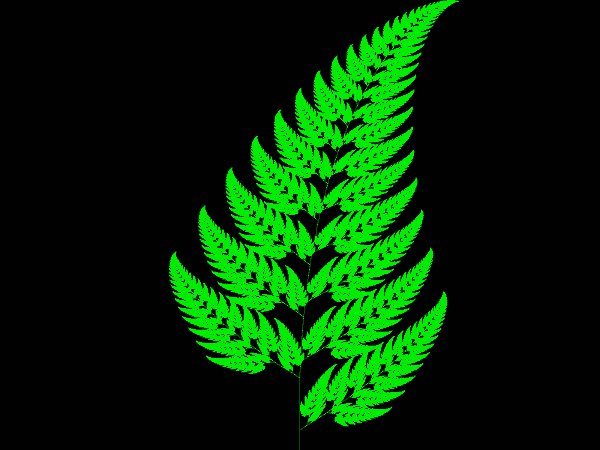We can then use different colors for each of the four functions to see which function colors what part by editing the above code as follows:
``````if(probability < 0.01) {
newpoint = f1.transform(point);
color = new Color(0xFF3800);
} else if(probability < 0.01 + 0.85) {
newpoint = f2.transform(point);
color = Color.GREEN;
} else if(probability < 0.01 + 0.85 + 0.07) {
newpoint = f3.transform(point);
color = new Color(0xFDEE00);
} else {
newpoint = f4.transform(point);
color = new Color(0x007FFF);
}
point = newpoint;``````
This produces the following output: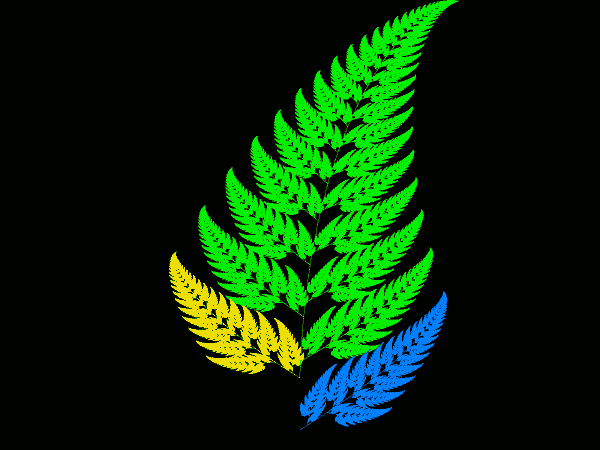We can play with colors and translation of point in other ways to render more aesthetic images.

### Mutations of the Barnsley Fern

The following are the mutations of the fern created by playing around with the values of the affine functions.
• Leptosporangiate fern
Functions a b c d e f Probability
f1 0.000 0.000 0.000 0.250 0.000 -0.14 0.020
f2 0.850 0.020 -0.02 0.830 0.000 1.000 0.840
f3 0.090 -0.28 0.300 0.110 0.000 0.600 0.070
f4 -0.09 0.280 0.300 0.090 0.000 0.700 0.070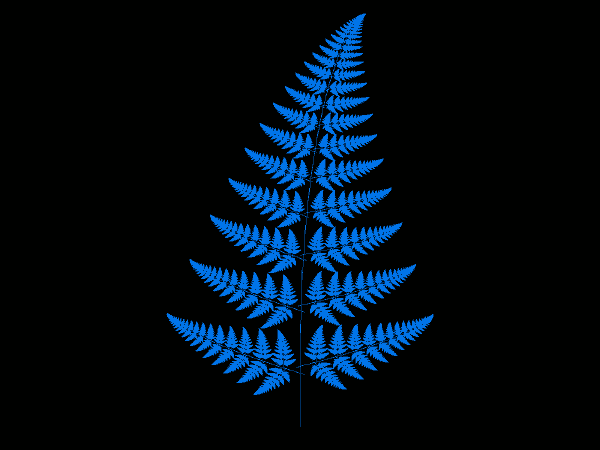• Fishbone Fern
Functions a b c d e f Probability
f1 0.000 0.000 0.000 0.250 0.000 -0.40 0.020
f2 0.950 0.002 -.002 0.930 -.002 0.500 0.840
f3 0.035 -0.11 0.270 0.010 -0.05 0.005 0.070
f4 -0.04 0.110 0.270 0.010 0.047 0.060 0.070• Thelypteridaceae fern
Functions a b c d e f Probability
f1 0.000 0.000 0.000 0.250 0.000 -0.40 0.010
f2 0.950 0.005 -.005 0.930 -.002 0.500 0.930
f3 0.035 -0.2 0.160 0.040 -0.09 0.020 0.030
f4 -0.04 0.200 0.160 0.040 0.083 0.120 0.030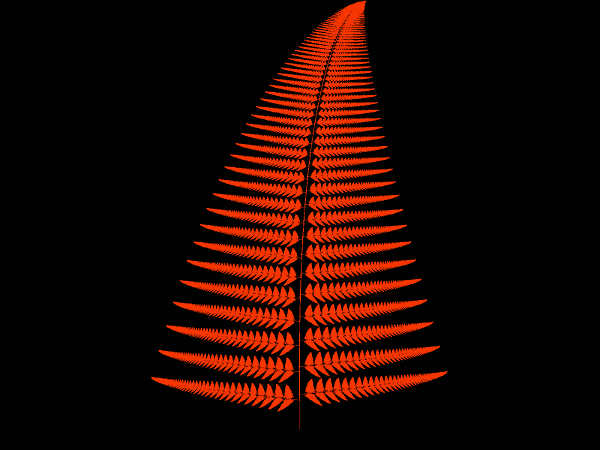Many more such mutations can be created by playing with the numbers.